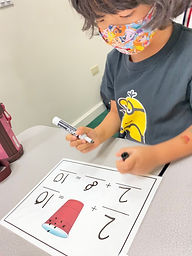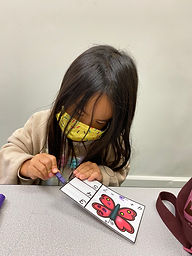## Ms. Brianne

### Target 1​

###### Lesson Type:

Review

Number Operation

:

Number System

Complete a number sequence from any given number 0 to 50.

###### 1:

Complete a number sequence in both ascending and descending order.

###### 2:

Start a number sequence from any given number.

Kindergarden

###### Vocabulary:

Number, Count on

Activities:

• Students counted to 50.
• Students played a race to 50 game. They took turns rolling a dice and coloring the matching number of squares until they reached 50. As they moved through the game, they practiced counting on and identifying each number they landed on.### Home Exploration

###### Guiding Questions:## Absent Students:

### Target 2

:

###### 1:

Use informal understandings of addition and subtraction to solve problems where one addend or subtrahend is given, and the sum or difference is given to solve for the unknown number.

Kindergarden

###### Vocabulary:

Unknown, Missing Number, Total

Activities:

• Students counted on to find the missing addend. After counting out the total with cubes, an unknown amount of the cubes were placed under a cup. They counted on and drew dots on a picture of a cup to help them determine the missing addend. Then they checked their answer by actually counting the cubes in the cup.
• Students were given an addition equation with a missing addend and a butterfly with dots on its wings to match one addend. Students added more dots to equal the total of the addition equation. Then they counted, wrote, and clipped the number of the missing addend.
• Students colored in a ten-frame to help them determine the missing addend to make 10.### Home Exploration

###### Guiding Questions:### Target 3

:

###### 1:

Looking at a picture where information is chaotic or spread out, count to gather data and answer questions.

###### 2:

Understand that data can be read more quickly in graph form.

Kindergarden

###### Vocabulary:

Data, Picture Graph

Activities:

• Students sorted shells according to color and organized them to create a picture graph.
• Students answered questions using the data in their graph.### Home Exploration